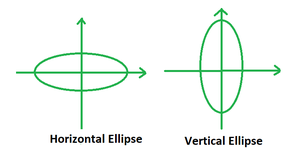GeeksforGeeks App
Open AppBrowser
Continue

# Find an equation for a horizontal ellipse with major axis that’s 50 units and a minor axis that’s 20 units

Conic sections also referred to as conics, are formed when a plane intersects a cone. The shape of such sections depends upon the angle at which the intersection happens. As such there are four types of conic sections, namely the circle, ellipse, parabola and hyperbola. Each of these shapes have their own properties and equations in mathematics. The ellipse is discussed below.

### Ellipse

An ellipse is a type of conic section which is formed when a plane intersects a cone at an angle of magnitude that lies between 0 and 90 degrees. As a result, it generalizes a circle, which is a special sort of ellipse with the same two focus points.The eccentricity of an ellipse, e, is a quantity ranging from e=0 to e=1 which shows how elongated it is along each axis. An ellipse has two axes, named the major axis and the minor axis. In the case of a horizontal ellipse, the axis along the x-axis would be called the major axis and the one along the y- axis is called the minor axis.

### Equation of an Ellipse

The standard form of the equation of an ellipse centred at the origin is written as follows:where a and b are the semi-major and minor axes respectively.

It is to be remembered that if a > b, then we have a horizontal ellipse on hand. Otherwise, if a < b, then it is a vertical ellipse.### Find an equation for a horizontal ellipse with a major axis that’s 50 units and a minor axis that’s 20 units.

Solution:

The standard form of equation of a horizontal ellipse with its center at the origin is, a > b.

Given: Length of major axis = 2a = 50 units

⇒ a = 50/2 = 25 units

Length of minor axis = 2b = 25 units

⇒ b = 20/2 = 10 units

Substituting the values in the equation, we have:### Similar Problems

Question 1. Make an equation for a horizontal ellipse with the major axis that’s 60 units and a minor axis that’s 20 units?

Solution:

The standard form of equation of a horizontal ellipse with its center at the origin is, a > b.

Given: Length of major axis = 2a = 60 units

⇒ a = 60/2 = 30 units

Length of minor axis = 2b = 20 units

⇒ b = 20/2 = 10 units

Substituting the values in the equation, we have:Question 2. Make an equation for a horizontal ellipse with the major axis that’s 30 units and a minor axis that’s 10 units?

Solution:

The standard form of equation of a horizontal ellipse with its center at the origin is, a > b.

Given: Length of major axis = 2a = 30 units

⇒ a = 30/2 = 15 units

Length of minor axis = 2b = 10 units

⇒ b = 20/2 = 5 units

Substituting the values in the equation, we have:Question 3. Make an equation for a horizontal ellipse with a major axis that’s 70 units and a minor axis that’s 40 units?

Solution:

The standard form of equation of a horizontal ellipse with its center at the origin is, a > b.

Given: Length of major axis = 2a = 70 units

⇒ a = 70/2 = 35 units

Length of minor axis = 2b = 40 units

⇒ b = 40/2 = 20 units

Substituting the values in the equation, we have:Question 4. Make an equation for a horizontal ellipse with a major axis that’s 100 units and a minor axis that’s 20 units?

Solution:

The standard form of equation of a horizontal ellipse with its center at the origin is, a > b.

Given: Length of major axis = 2a = 100 units

⇒ a = 100/2 = 50 units

Length of minor axis = 2b = 20 units

⇒ b = 20/2 = 10 units

Substituting the values in the equation, we have:Question 5. Make an equation for a horizontal ellipse with a major axis that’s 42 units and a minor axis that’s 6 units?

Solution:

The standard form of equation of a horizontal ellipse with its center at the origin is, a > b.

Given: Length of major axis = 2a = 42 units

⇒ a = 42/2 = 21 units

Length of minor axis = 2b = 6 units

⇒ b = 6/2 = 3 units

Substituting the values in the equation, we have:My Personal Notes arrow_drop_up
Related Tutorials